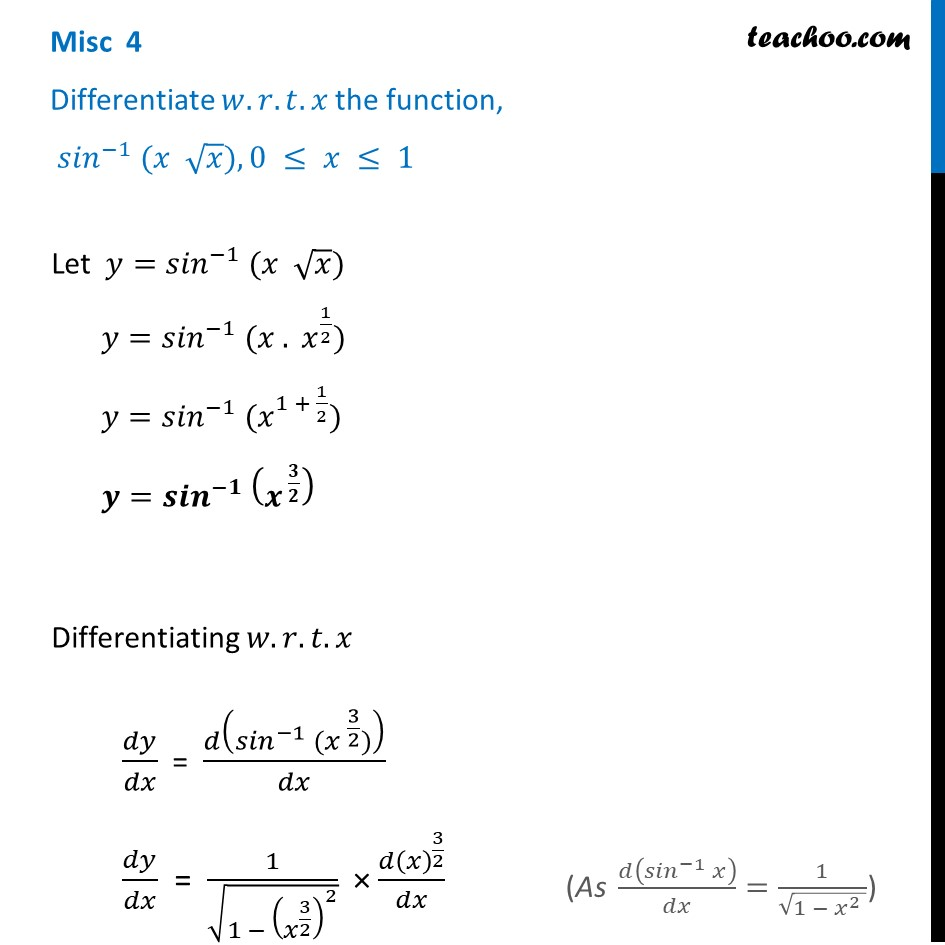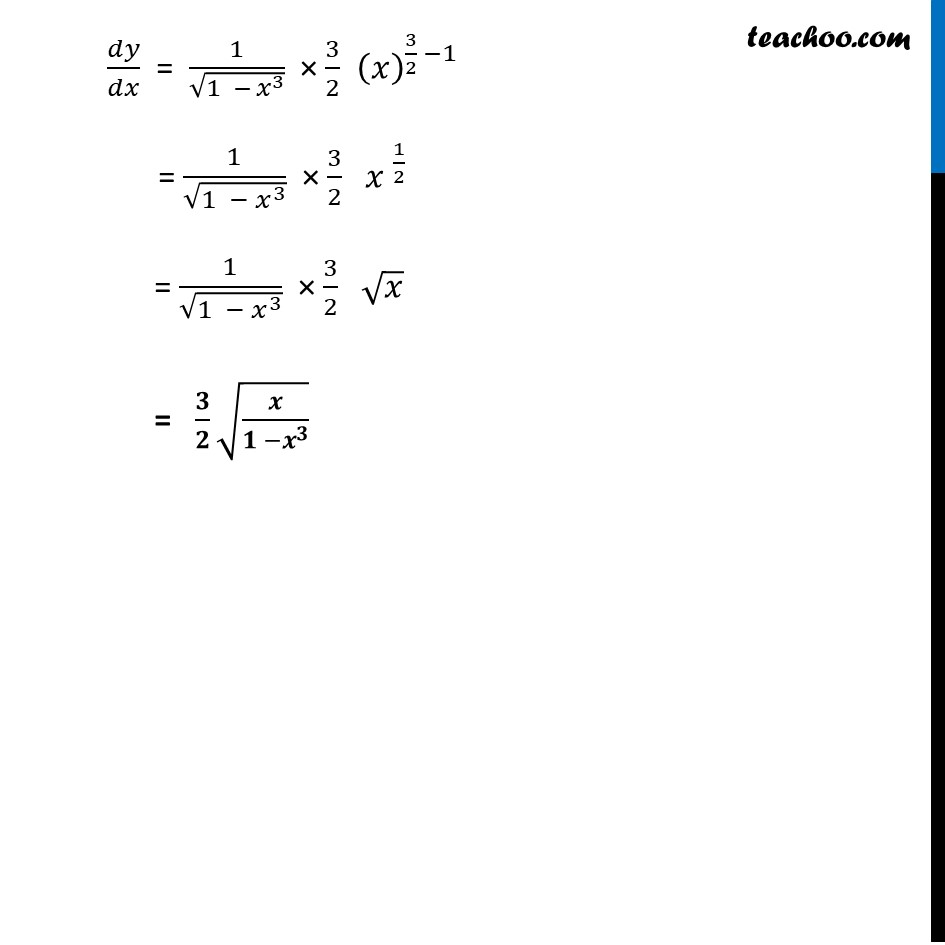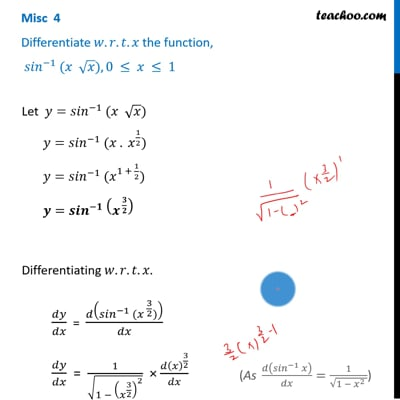Finding derivative of Inverse trigonometric functions

Chapter 5 Class 12 Continuity and Differentiability
Concept wiseThis video is only available for Teachoo black users

Introducing your new favourite teacher - Teachoo Black, at only ₹83 per month

### Transcript

Misc 4 Differentiate 𝑤.𝑟.𝑡. 𝑥 the function, 〖𝑠𝑖𝑛〗^(−1) (𝑥 √𝑥), 0 ≤ 𝑥 ≤ 1 Let 𝑦=〖𝑠𝑖𝑛〗^(−1) (𝑥 √𝑥) 𝑦=〖𝑠𝑖𝑛〗^(−1) (𝑥 . 𝑥^(1/2)) 𝑦=〖𝑠𝑖𝑛〗^(−1) (𝑥^(1 + 1/2)) 𝒚=〖𝒔𝒊𝒏〗^(−𝟏) (𝒙^( 𝟑/𝟐) ) Differentiating 𝑤.𝑟.𝑡. 𝑥 𝑑𝑦/𝑑𝑥 = 𝑑(〖𝑠𝑖𝑛〗^(−1) (𝑥^( 3/2)))/𝑑𝑥 𝑑𝑦/𝑑𝑥 = 1/√(1 − (𝑥^(3/2) )^2 ) × (𝑑(𝑥)^(3/2))/𝑑𝑥 ("As " 𝑑(〖𝑠𝑖𝑛〗^(−1)⁡𝑥 )/𝑑𝑥=1/√(1 − 𝑥^2 )) 𝑑𝑦/𝑑𝑥 = 1/√(1 − 𝑥^3 ) × 3/2 (𝑥)^(3/2 −1) = 1/√(1 − 𝑥^3 ) × 3/2 〖𝑥 〗^(1/2 ) = 1/√(1 − 𝑥^3 ) × 3/2 √𝑥 = 𝟑/𝟐 √(𝒙/(𝟏 −𝒙^𝟑 ))